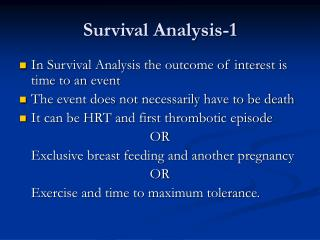# Survival Analysis-1 - PowerPoint PPT PresentationDownload PresentationSurvival Analysis-1

Survival Analysis-1Download Presentation## Survival Analysis-1

- - - - - - - - - - - - - - - - - - - - - - - - - - - E N D - - - - - - - - - - - - - - - - - - - - - - - - - - -
##### Presentation Transcript

1. Survival Analysis-1 • In Survival Analysis the outcome of interest is time to an event • The event does not necessarily have to be death • It can be HRT and first thrombotic episode OR Exclusive breast feeding and another pregnancy OR Exercise and time to maximum tolerance.

2. Survival Analysis-2 Studying time to an event poses two difficulties: • Time interval can vary from one subject to another. Also at the end of the study the event may not have occurred for many subjects. So time to event is not Normally distributed. • In a long period of follow-up many subjects drop out. The only information we have about them is until last follow-up. These are Censored Observations.

3. Assumptions in Survival Analysis • Survival prospects are assumed to stay the same throughout the study. • Subjects lost to follow-up have the same prognosis as those who continue with the study. • The probability that an individual is censored is unrelated to the probability that the individual suffers an event. • Anything which affects the hazard does so by the same proportion at all time.

4. Calculating Kaplan –Meier Survival Curve At the start of the study (t0), proportion surviving = 1. Order survival time by increasing duration starting with the shortest. At each time interval from 1 to n survival time is calculated by S (ti) = ( ri – di / ri ) x S (ti -1) Where di = number of events at time ti For censored observations di = 0. ri = number alive just before it. For censored observations survival curve remains unchanged. At the next event the number ‘at risk’ is reduced by the number censored between the two events.

5. Hazard Curve H0 is the baseline hazard at time t i.e. h0(t) For any individual subject the hazard at time t is hi(t). Hi(t) is linked to the baseline hazard h0(t) by loge{hi(t)} = loge{h0(t)} + β1X1 + β2X2 +……..+ βpXp where X1, X2 and Xp are variables associated with the subject. Then hi(t) = h0(t) eβ1X1 + β2X2 +….+ βpXp H0(t) represents the hazard for an individual where X1=X2=Xp=0. h(t) / h0(t) depends on the predictor variables and not on t h(t) / h0(t) is the hazard ratio

6. Data Analysis • The study to be analysed is about comparing survival time of 49 patients with colorectal cancer after random allocation to γ linoleic acid and controls

7. Results - 1 Standard 95.0% Normal CI Mean(MTTF) Error Lower Upper 23.7425 3.0506 17.7634 29.7216 Median = 24.0000 IQR = 23.0000 Q1 = 13.0000 Q3 = 36.0000 Kaplan-Meier Estimates Number Number Survival Standard 95.0% Normal CI Time at Risk Failed Probability Error Lower Upper 1.000 25 1 0.9600 0.0392 0.8832 1.0000 5.0000 24 1 0.9200 0.0543 0.8137 1.0000 9.0000 21 1 0.8762 0.0671 0.7447 1.0000 10.0000 20 1 0.8324 0.0767 0.6821 0.9827 12.0000 17 1 0.7834 0.0864 0.6140 0.9528 13.0000 12 1 0.7181 0.1009 0.5204 0.9159 Mean Time to Failure is the measure of the average survival time The Median gives the survival time for 50% of the subjects followed by survival probability table.

8. Results - 2 Comparison of Survival Curves Test Statistics Method Chi-Square DF P-Value Log-Rank 0.06986 1 0.7915 Wilcoxon 0.7048 1 0.4012 There is no significant difference in survival between the two groups.